# Points, lines, and angles worksheet (with answer key)

One of geometry’s fundamental forms is a line. Different points can make a line. A line can also make an angle. Use this math worksheet to help you identify points, lines, and angles.

## What is the “Points, lines, and angles worksheet”?

Points, lines, and angles worksheet is a math practice sheet that incorporates reflection, application, and problem-solving with a challenge to aid students in creating and resolving their word problems. This activity will assist students in understanding the lecture, applying new knowledge, and utilizing prior knowledge.

This worksheet will help you learn the points, lines, and angles and how to identify them.

## Instructions on how to use the “Points, lines, and angles worksheet.”

Use this math worksheet to explore points, lines, and angles in detail and learn how to identify them. We provide a 5-item activity to help you practice the topic.

.

The worksheet’s last part, titled “Reflection,” invites students to assess their learning throughout the lecture and their metacognition.

### Conclusion

Points, lines, and angles are simple as long as you remember what they look like and are familiar with their definition.

## Note to Remember:

• A dot can be used to symbolize a position as a point. A point has a position; it has no length, width, shape, or size.
• A line is formed when two separate points are joined.
• Acute angles are less than 90 degrees.
• The right angles are 90 degrees.
• Obtuse angles are greater than 90 degrees.
• Straight angles are 180 degrees.
• The complementary angle has a sum of 90 degrees.
• Supplementary angles have a sum of 180 degrees.

Identification:

Write A if the figure is an angle and determine what kind of angle it is. Write L if it is a line and P if it is a point.

1.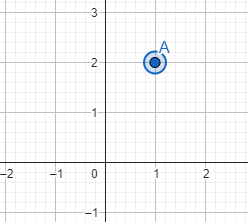2.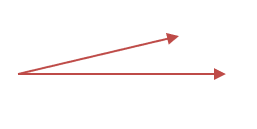3.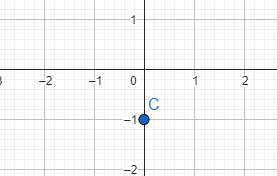4.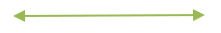5.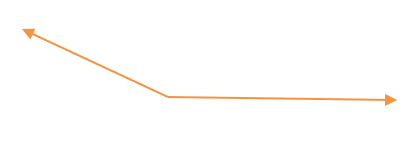Reflection:

What do you think about points, lines, and angles? Did you find it challenging and hard to solve them?

Real-Life Applications

Draw an object that has a point, line, and angle that you can see in real life. For example, a clock.